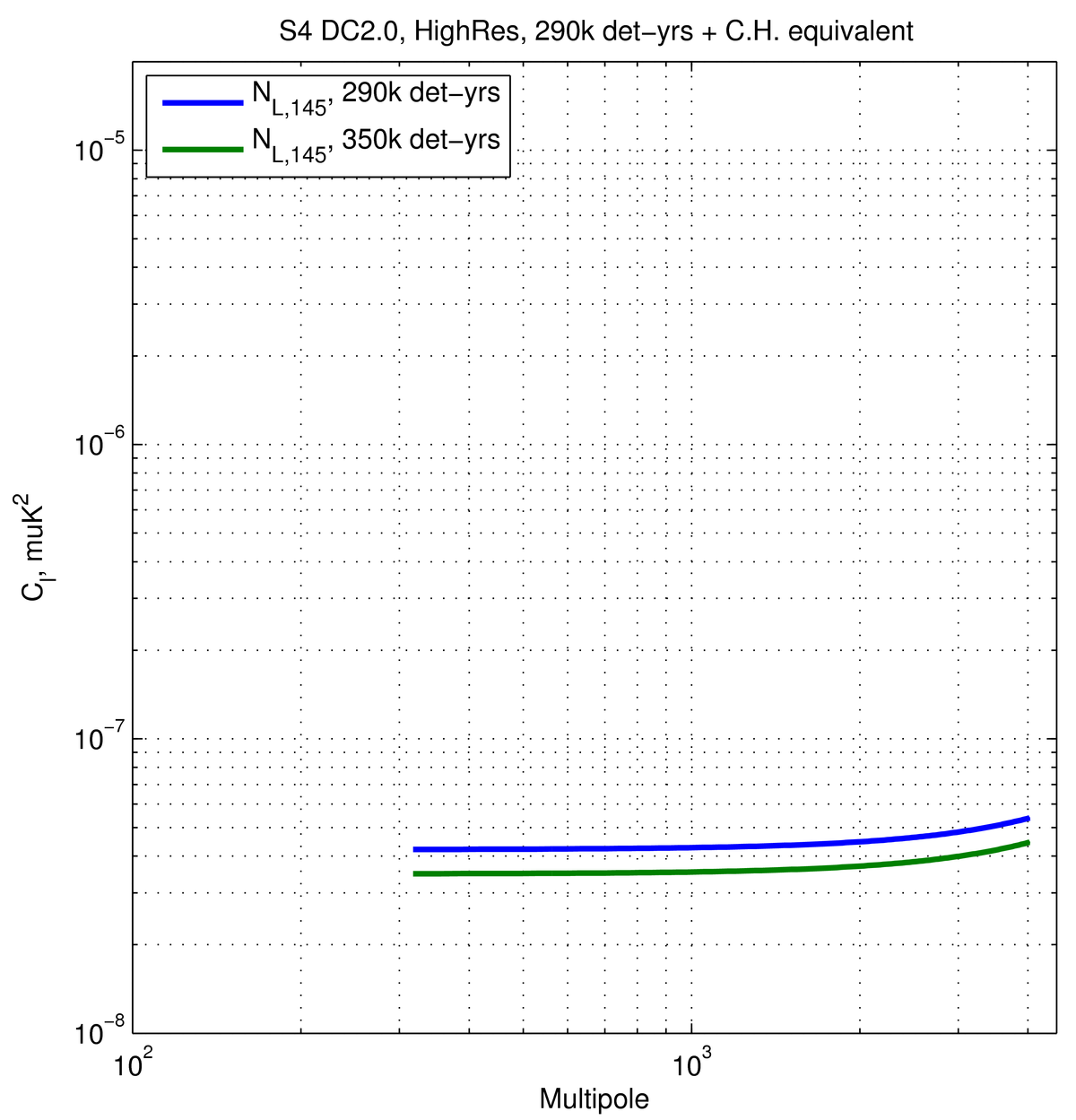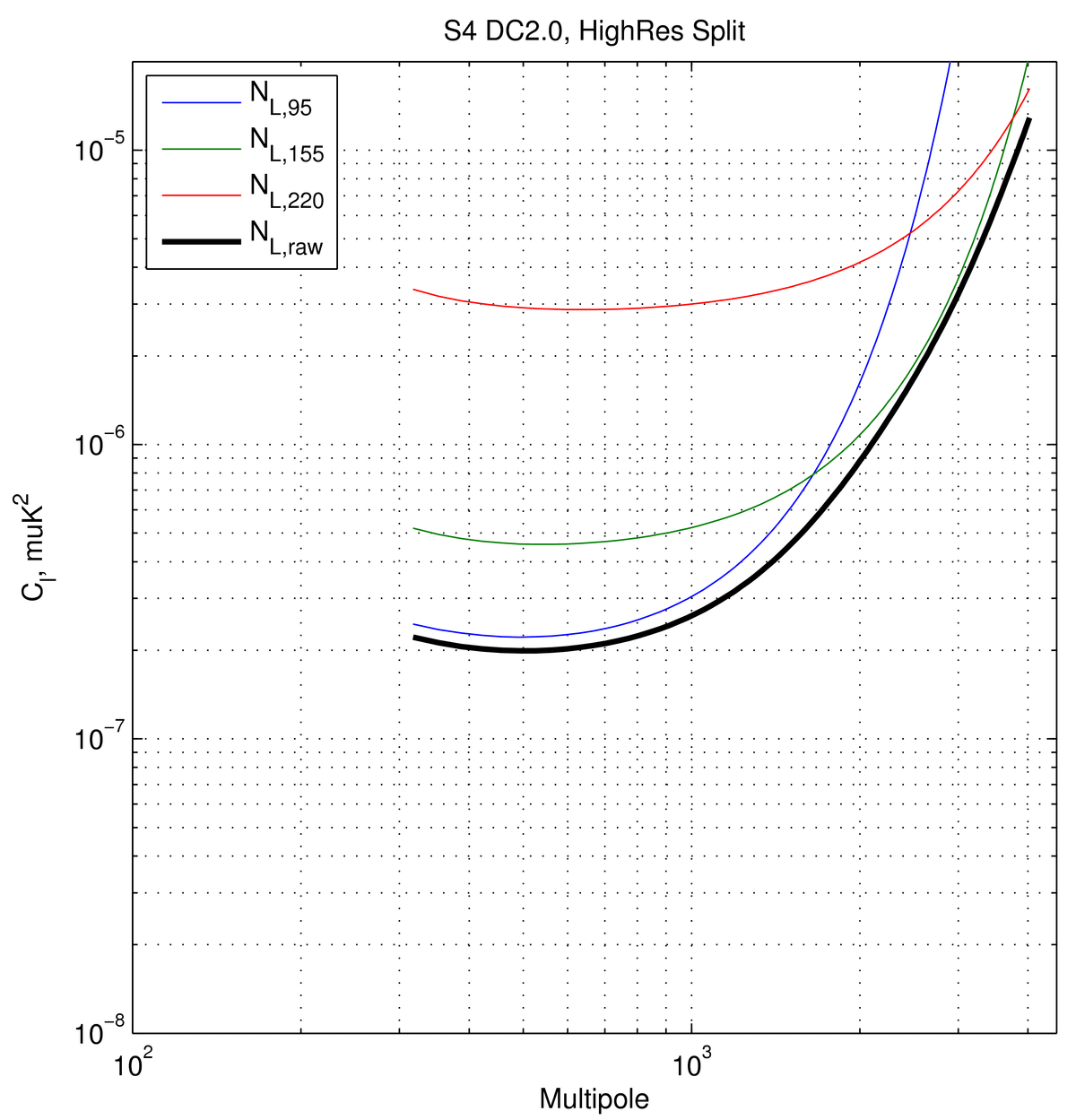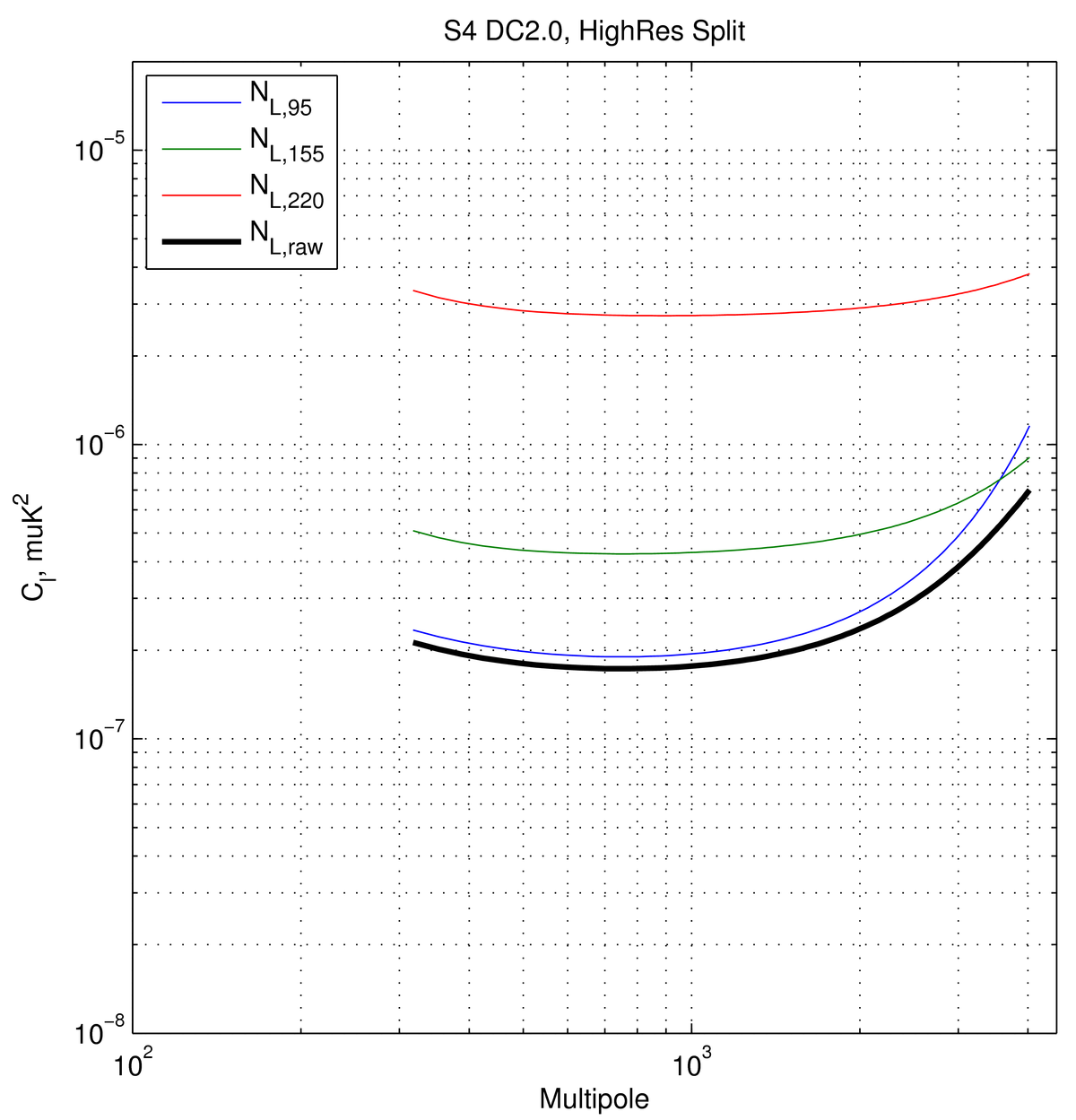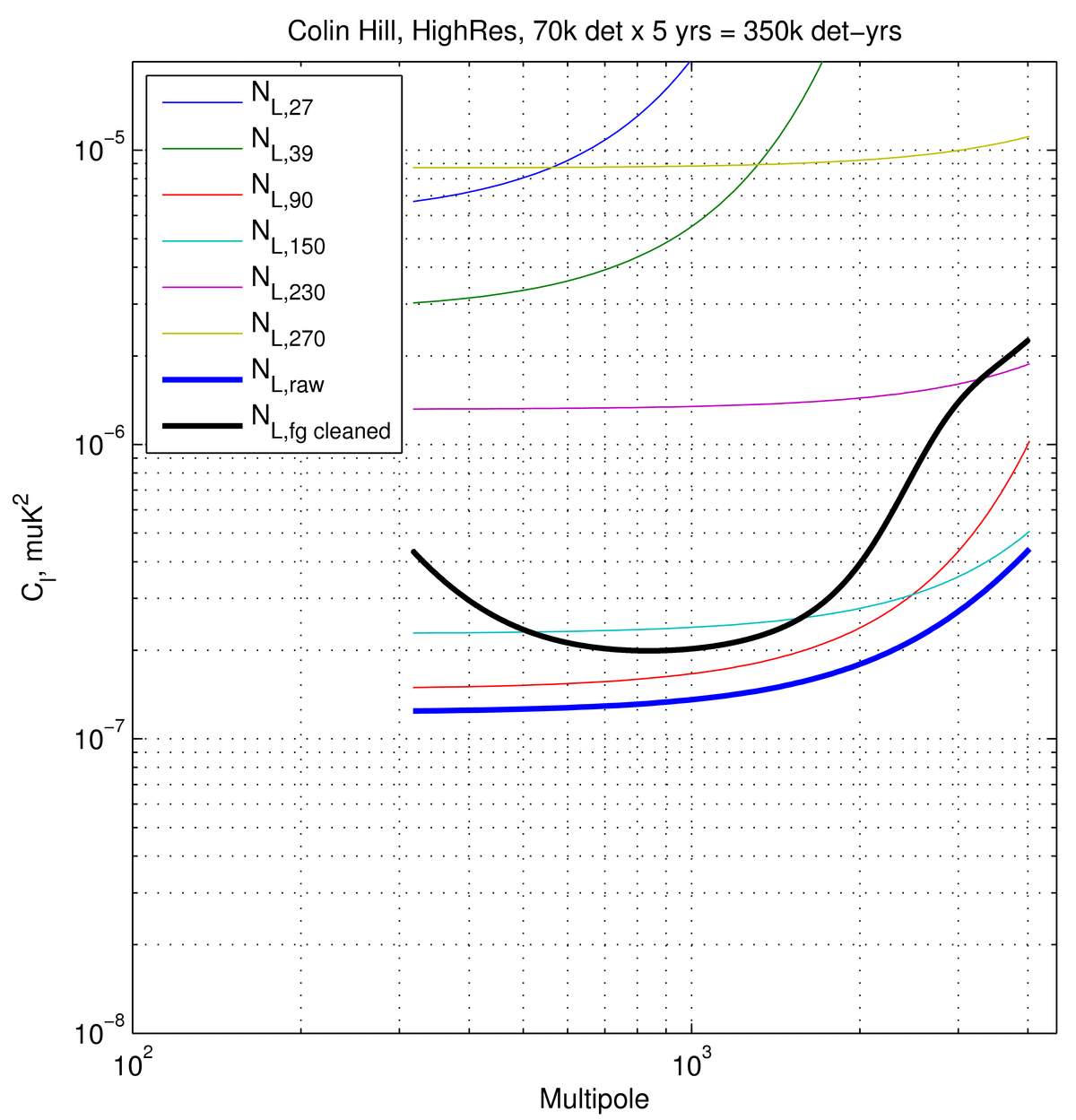High-Res studies for CMB-S4 (draft, v2)

2017-08-18  Victor Buza

There have been many postings describing the optimization that went into the Science Book and subsequently in the Data Challenges. For a comprehensive list of such write-ups please take a look here. In this posting, I first describe in more detail how the HighRes side of the optimization is handled and derive delensing factors for some specific cases; I then take a look at the HighRes setups for DC2.0 and DC3.0 as well as a particular HighRes setup coming from Colin Hill (for SO) and compute delensing factors for these cases as well.

1. HighRes Setup for Science Book Optimization

We have now gone through a few iterations of the the optimization. For each case, I produce a posting that describes the optimization, and one that offers analytic fitting parameters for constructing $$N_{l}$$ curves that are fitted to the real $$N_l$$'s (scaled for appropriate effort levels) and lists the fraction of effort spent towards solving the foreground separation problem ("degree-scale effort") and reducing the lensing contribution ("arcmin-scale effort").

For the arcminute scale effort, as described here, to calculate an effective level of residual lensing, an experiment with $$1$$ $$arcmin$$ resolution, and mapping speed equivalent to the 145 LowRes channel was assumed. In particular, this procedure takes into account achieved on sky detector performance (yield, mapping speed, effective observing time, etc), but unlike the LowRes part, it does not account for mode filtering, which affects sky coverage and S/N per mode, or for noise contributions that take into account the non-uniformity of our surveys. Not taking into account these effects results into a uniform survey-weight and no reduction in the effective numbers of degrees-of-freedom due to filtering -- equivalently it results in deeper maps. One can argue wether this is the right thing to do when using LowRes information to inform a HighRes experiment. To quantify this effect, in addition to what was done for the Science Book, below I also look at taking filtering and survey non-uniformity into account for the HighRes experiment -- this gets the moniker "HighRes (+terms)."

Using an iterative estimator (code developed by Kimmy Wu), following the formalism in Smith et al. (arXiv: 1010.0048), a $$C_{\ell, res}/C_{\ell, lens}$$ is calculated, the results of which are presented in this plot. PR stands for the experiment used for "phi/lensing reconstruction," and EM stands for the experiment (or combination of experiments) used for getting the E-modes. The combined map-depth of EM is assumed to be $$1 \mu K$$-arcmin, though we've seen before (from Kimmy) that the ratio of $$C_{\ell, res}/C_{\ell, lens}$$ depends quite little on this noise, as seen here. The $$l_{min}$$ in the plot is for the E/B inputs to $$\Phi.$$ For all optimizations I assume $$l_{min,PR}=200$$, $$l_{max}=4000$$, and all cases assume complete E-mode coverage (i.e. $$l_{min,EM}=30$$) for the formation of the B-mode template. Practically, this is a scenario in which the arcmin-scale experiment may be noisy at low $$l$$, but we can nonetheless measure all of the E-modes through this range to the level of precision required either with the arcmin-scale or degree-scale experiments. This complete E-mode map is then used to form a B-template by lensing these E-modes with the reconstructed $$\Phi$$.

Below is a worked example for the case presented here.

Figure 1:

Left: $$N_{l}$$'s for the Degree Scale effort that went into Data Challenges 2.0 and 3.0, amounting to ~575k actual det-yrs on $$f_{sky}=0.03$$, as recorded here

Middle: $$N_{l}$$'s for the Arminute Scale effort that went into the optimization for DC2.0 and DC3.0, amounting to ~290k actual det-yrs (at 145 GHz) on $$f_{sky}=0.03$$, as recorded here. For the data challenges, this effort was ultimately split into three bands -- see next section for details. The high-res details are recorded above.

In addition, I also add a 350k det-yr case for comparison with the plot in the last section.

Right: $$N_{l}$$'s for the Arcminute Scale effort that went into the the optimization for DC2.0 and DC3.0, taking into account filtering and survey non-uniformity (described above). These curves are effectively directly scaled-down versions of the yellow or black curves in the left-most panel (after accounting for beam factors and the presence of 1/f noise in the LowRes panel).

Note: One can note the difference between the "HighRes" and "HighRes (+terms)" noise curves, and that the difference results, for this particular case, in a 4% increase in residual lensing power.

Table 1:
Effective $$A_L$$ levels derived using the assumptions described above and the noise curves below.
HighResHighRes (+terms)
290k det-yrs$$A_L=0.09$$$$A_L=0.13$$
350k det-yrs$$A_L=0.08$$$$A_L=0.12$$2. HighRes Setup for DC2.xx/3.xx

Figure 2:

For DC2.0 and DC3.0 the HighRes effort was split into three bands. The split was motivated by the LowRes optimization -- i.e. the $$f_{sky}=0.03$$ entry in the table in this posting. This results in putting 60.0% of the effort at 95, 21.6% at 150, and 18.4% at 220 (after normalizing to 100%). That means 310,000 det-yrs (150-equiv) get divided into (186,000; 66,960; 57,040) det-yrs 150 equiv. This gives us (1.40, 2.19, 5.61) uK-arcmin at (95, 155, 220) for the HighRes part.

In addition to white noise levels, as we see in the formula in this posting, we have an $$l_{knee}=200$$ with slope $$\gamma=-2.0$$ for 95 and $$\gamma=-3.0$$ for 150 and 220. For this data challenge the beams were chosen by Clem to be {6.1, 4.0, 2.7} arcmin for {95, 155, 220} respectively. I believe this is for a roughly 3 meter aperture scaled from BICEP3. Below, I also consider a 5 meter dish yielding resolutions of {2.8, 1.8, 1.2} arcmin (equivalent to the SO resolutions for these frequencies).

These details are recorder in the S4 Experiment Definitions section.

Note: There are two reasons that yield a larger lensing residual here when compared to the first section. First, the larger beams play a role, as evidenced by the fact that a larger dish improves the delensing efficiency. Second, splitting the effort into three frequencies results in a higher $$N_{raw}$$ than if one were to lump all the effort at 155 for instance. One can see that by taking the green curve, which is for ~67k det-yrs (150 equiv), and scaling down by 310/67=4.6 under the assumption that all ~310k det-yrs (150 equiv) are at 155 now, and comparing to the blue line in the right-most plot above. Both are below the solid black line here.

Table 2:
Effective $$A_L$$ levels derived using the assumptions described above and the noise curves below.
HighRes, Small DishHighRes, Large Dish
290k det-yrs$$A_L=0.26$$$$A_L=0.20$$3. HighRes Setup for SO

Figure 3:

Another set of HighRes inputs comes from the SO working group, as presented here. This posting assumes an optimized 5 meter dish given a particular choice of foreground model, frequency selection, and detector performance. The closest case presented is a case with 70k det-yrs x 5 yrs = 350k det-yrs, on $$f_{sky}=0.03$$. The individual $$N_l$$ curves are formed from the white noise and beam widths offered, while the blue solid curve is the combined raw noise level, and the black solid curve is the resulting foreground cleaned $$N_l$$.

Note: The ILC method used here to clean foregrounds tries to completely zero out the foreground contributions both at low and high $$l$$, resulting in higher noise in the synthesized CMB channel. As per Tom Crawford's suggestion, this should be considered a pretty conservative approach. In the absence of foregrounds, all the effort could be lumped into one channel, such as 150 GHz for instance, giving us a new $$N_{raw}$$ curve that would be roughly 3 times lower than the cyan one (assuming 1/3 of the effort is currently at 150), yielding a foreground degradation factor (when compared to the solid black) of roughly 3 at $$l=1000$$, 4.5 at $$l=2000$$, and 13.5 at $$l=3000$$.

Keeping in mind the points above, as well as the points made in Section 1 about LowRes to HighRes translation, one can compare the resulting $$A_L$$'s in the Table below with the second row of Table 1, as well as compare the $$A_L$$'s between the raw noise and fg cleaned noise choices.

Table 3:
Effective $$A_L$$ levels derived using the assumptions described above and the noise curves below.
$$N_{l,raw}$$$$N_{l,fg cleaned}$$
350k det-yrs$$A_L=0.17$$$$A_L=0.23$$